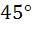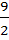Through the point (3, 4) are drawn two straight lines each inclined atto the straight line x − y = 2. Find their equations and find the area included by the three lines.
 My Self Assessment CLEAR « Previous Answer:a) Equation of PR is y = 4 and equation of AB is x − y = 2; area of △PQR issquare units. Next »